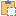#### AnalyticalModelSelector Class

Defines a portion of an Analytical Model for an Element.

Namespace: Autodesk.Revit.DB.Structure
Assembly: RevitAPI (in RevitAPI.dll) Version: 17.0.0.0 (17.0.484.0)
Since: 2011

# Syntax

C#
``public class AnalyticalModelSelector : IDisposable``
Visual Basic
``````Public Class AnalyticalModelSelector _
Implements IDisposable``````
Visual C++
``public ref class AnalyticalModelSelector : IDisposable``

# Remarks

This is used to identify the portion of an analytical model of interest to a client. To identify the portion of the analytical model, the client must identify the curve in question, by using one of the following:
• The specific curve within the analytical model.
• The index of the curve within the analytical model.
If a specific part of that curve is of interest, the client needs to identify that by specifying one of the following:
• The start of the curve.
• The end of the curve.
• The entire curve. This is the default behavior, so this does not need specifying.

# ExamplesCopy C#
``````public bool GetReferenceData(FamilyInstance familyInst)
{
AnalyticalModel analyticalModelFrame = familyInst.GetAnalyticalModel();
Curve analyticalCurve = analyticalModelFrame.GetCurve();
if (null != analyticalCurve)
{
// test the stable reference to the curve.
AnalyticalModelSelector amSelector = new AnalyticalModelSelector(analyticalCurve);
amSelector.CurveSelector = AnalyticalCurveSelector.WholeCurve;
Reference curveReference = analyticalModelFrame.GetReference(amSelector);

// test the stable reference to the start point of the curve
amSelector.CurveSelector = AnalyticalCurveSelector.StartPoint;
Reference startPointReference = analyticalModelFrame.GetReference(amSelector);

// test the stable reference to the start point of the curve
amSelector.CurveSelector = AnalyticalCurveSelector.EndPoint;
Reference endPointReference = analyticalModelFrame.GetReference(amSelector);
}

return true;
}``````Copy VB.NET
``````Public Function GetReferenceData(familyInst As FamilyInstance) As Boolean
Dim analyticalModelFrame As AnalyticalModel = familyInst.GetAnalyticalModel()
Dim analyticalCurve As Curve = analyticalModelFrame.GetCurve()
If analyticalCurve IsNot Nothing Then
' test the stable reference to the curve.
Dim amSelector As New AnalyticalModelSelector(analyticalCurve)
amSelector.CurveSelector = AnalyticalCurveSelector.WholeCurve
Dim curveReference As Reference = analyticalModelFrame.GetReference(amSelector)

' test the stable reference to the start point of the curve
amSelector.CurveSelector = AnalyticalCurveSelector.StartPoint
Dim startPointReference As Reference = analyticalModelFrame.GetReference(amSelector)

' test the stable reference to the start point of the curve
amSelector.CurveSelector = AnalyticalCurveSelector.EndPoint
Dim endPointReference As Reference = analyticalModelFrame.GetReference(amSelector)
End If

Return True
End Function``````

# Inheritance Hierarchy

System Object
Autodesk.Revit.DB.Structure AnalyticalModelSelector